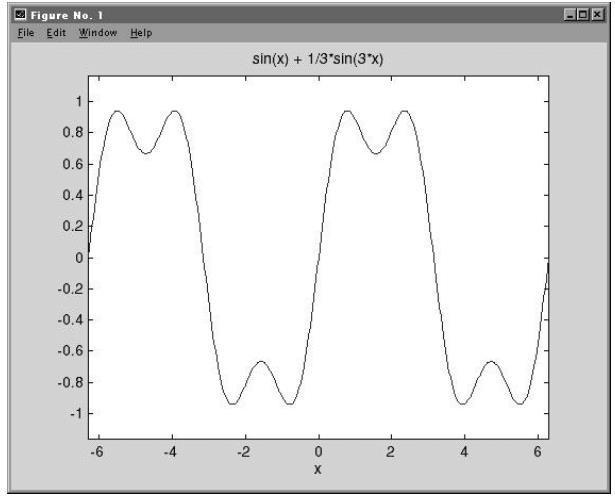# The Example of Working with the Symbolic Toolbox in the MATLAB

Task: get basic knowledge of how to work with the symbolic toolbox in the MATLAB software package (Part 1).

Solution:

MATLAB is probably best known for its capability to perform numerical computations. This is particularly true when you consider the native capability of MATLAB itself. With the addition of the Symbolic Math Toolbox included in the student edition and the Extended Symbolic Math Toolbox, however, MATLAB can perform true symbol computations. MATLAB achieves its symbolic capability via the symbolic processor engine.

The Maple engine provides many capabilities, such as:

• factor, simplify, etc.
• equation solving
• solve differential equations
• differentiation
• integration
• linear (matrix) algebra
• fourier, Laplace, and z-transforms
• numerical evaluation of expressions to a specified accuracy

Symbolic Expressions

A symbolic expression in MATLAB is created as a character string using the function sym(S) where S is a string:

S = sym(‘sin(x + z)’);

S = sym(‘2*x^3 + x’);

S = sym(‘1/(cos(x) + angle)’);

S = sym(‘exp(x^2)’);

When symbolic expressions are created as in the above examples, there may be more than one variable present.

To find the variables in a symbolic expression or matrix, we can use the function findsym(S):

S = sym(‘sin(x + z)’);
findsym(S) ans = x, z % Finds all variables
findsym(S,1) ans = x % Finds the one variable closest to x

The variation findsym(S,n) finds the n variable names closest to the letter x.

If we wish to create some working variables, as opposed to expressions, we can use the shorthand function syms:

a = sym(‘a’);

b = sym(‘b’);

x = sym(‘x’);

y = sym(‘y’);

This is a lot of typing, so instead you can use:

syms a b x y

To see what this is doing, type whos:

S = sym(‘sin(x + z)’);
syms a b x y
whos

Name Size Bytes Class

S 1×1 144 sym object

a 1×1 126 sym object

b 1×1 126 sym object

x 1×1 126 sym object

y 1×1 126 sym object

Grand total is 19 elements using 648 bytes

We see that we have created sym(bolic) objects. The ability to create objects as opposed to just plain variables is a new feature in MATLAB 5, hence the differences between symbolic operations.

Once a symbolic expression is defined using sym(S) we can take advantage of some powerful symbolic toolbox features, such as ezplot:

– ezplot(S) plots the expression in S on the interval to assuming it contains only one variable

– ezplot(S,[xmin,xmax]) plots the expression in S on the specified interval

For example:

S = sym(‘sin(x) + 1/3*sin(3*x)’);
ezplot(S)Another example:

S1 = x^3 – 1

S2 = (x – 3) ^2* (y – 4) ^2

S1 = sym(‘x^3 – 1’);

S2 = sym(‘(x-3) ^2 + (y-4) ^2’);

Alternate construction

syms x y S1 = x^3 – 1;

S2 = (x-3) ^2 + (y-4) ^2;

% Now perform some simplifications

factor(S1)

ans = (x-1) * (x^2+x+1)

% Try the pretty form
help pretty %

PRETTY Pretty prints the Maple string.

PRETTY(S) prints the Maple string in a format that resembles type-set mathematics.

PRETTY(S,n) uses screen width n instead of the default 79.

%%%%

pretty(factor(S1))

2

(x – 1) (x + x + 1)

pretty(expand(S2))

2

2 x – 6 x + 25 + y – 8 y

pretty(collect(S2))

2

2 x – 6 x + 9 + (y – 4)

Many students who study engineering can find this guide very helpful. Nevertheless, we can offer you not only samples, but assistance proved by experts in the field. Our purpose is to help students with any homework problem. We give you the opportunity to deal with your homework faster and easier. When you place an order on our site, don’t forget to mention your requirements, provide information that you want to be used in the assignment and set the deadline. What is more important is that you can choose an expert on your own after they out bids on your order.

You can contact us any time you want – we work 24/7. In order to get a completed assignment that you want, include all the instructions. A huge number of students have already used our service and remain satisfied with it. If you want to be one of those students, visit https://assignmentshark.com/.